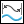Length—Measures the length of a curve, edge, or perimeter.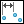Distance—Measures the distance between two reference entities.Angle—Measures the angle between two reference entities.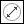Diameter—Measures the diameter of a surface.Area—Measures the area of a surface, quilt, facet, body, or model.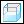Volume—Measures the volume of a body, model, or closed quilt. Measures the volume of a body or model on one side of a designated plane.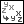Transform—Measures the transformation matrix between two selected coordinate systems.